# MAFS.4.NF.1.1Archived StandardExport Print
Explain why a fraction a/b is equivalent to a fraction (n × a)/(n × b) by using visual fraction models, with attention to how the number and size of the parts differ even though the two fractions themselves are the same size. Use this principle to recognize and generate equivalent fractions.

### Remarks

Examples of Opportunities for In-Depth Focus

Extending fraction equivalence to the general case is necessary to extend arithmetic from whole numbers to fractions and decimals.
General Information
Subject Area: Mathematics
Domain-Subdomain: Number and Operations - Fractions
Cluster: Level 3: Strategic Thinking & Complex Reasoning
Cluster: Extend understanding of fraction equivalence and ordering. (Major Cluster) -

Clusters should not be sorted from Major to Supporting and then taught in that order. To do so would strip the coherence of the mathematical ideas and miss the opportunity to enhance the major work of the grade with the supporting clusters.

Date of Last Rating: 02/14
Status: State Board Approved - Archived
Assessed: Yes
Test Item Specifications

• Assessment Limits :
Denominators of given fractions are limited to: 2, 3, 4, 5, 6, 8, 10, 12, 100. For items with denominators of 10 and 100, focus may not be on equivalence between these 2 denominators, since this is addressed specifically in standards MAFS.4.NF.5 – 7, but should focus on equivalence between fractions with denominators of 2, 4, and 5, and fractions with denominators of 10 and 100, e.g.,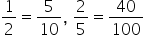, etc.

Fractions must refer to the same whole, including in models. Fraction models are limited to number lines, rectangles, squares, and circles. Fractions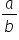can be fractions greater than 1 and students may not be guided to put fractions in lowest terms or to simplify. Equivalent fractions also include fractions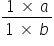.

• Calculator :

No

• Context :

Allowable

Sample Test Items (3)
• Test Item #: Sample Item 1
• Question:

Select all the models that have been shaded to represent fractions equivalent to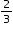• Difficulty: N/A
• Type: MS: Multiselect

• Test Item #: Sample Item 2
• Question:

Corey tried to find a fraction equivalent to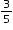. His work is shown.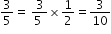Which statement describes Corey's error?

• Difficulty: N/A
• Type: MC: Multiple Choice

• Test Item #: Sample Item 3
• Question:

Kari represented a fraction by shading parts of the model shown.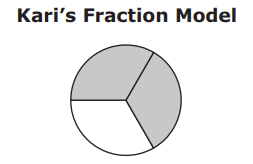Select all the models that have been shaded to represent fractions equivalent to Kari’s fraction.

• Difficulty: N/A
• Type: MS: Multiselect

## Related Courses

This benchmark is part of these courses.
5012060: Mathematics - Grade Four (Specifically in versions: 2014 - 2015, 2015 - 2022, 2022 and beyond (current))
7712050: Access Mathematics Grade 4 (Specifically in versions: 2014 - 2015, 2015 - 2018, 2018 - 2022, 2022 and beyond (current))
5012055: Grade 3 Accelerated Mathematics (Specifically in versions: 2019 - 2022, 2022 and beyond (current))
5012015: Foundational Skills in Mathematics 3-5 (Specifically in versions: 2019 - 2022, 2022 and beyond (current))

## Related Access Points

Alternate version of this benchmark for students with significant cognitive disabilities.

## Related Resources

Vetted resources educators can use to teach the concepts and skills in this benchmark.

## Formative Assessments

Equivalent Fractions on a Number Line:

Students scale number lines to locate given fractions, find equivalent fractions, and explain the relationship between equivalent fractions.

Type: Formative Assessment

Equivalence Using A Number Line:

Students use a number line to explain that one-half is equivalent to two-fourths.

Type: Formative Assessment

Eating Cake:

Students draw a visual fraction model to determine whether two fractions are equivalent.

Type: Formative Assessment

Are the Fractions Equivalent:

Students partition squares to model two fractions and then determine if the fractions are equivalent.

Type: Formative Assessment

## Image/Photograph

Clipart ETC Fractions:

Illustrations that can be used for teaching and demonstrating fractions. Fractional representations are modeled in wedges of circles ("pieces of pie") and parts of polygons. There are also clipart images of numerical fractions, both proper and improper, from halves to twelfths. Fraction charts and fraction strips found in this collection can be used as manipulatives and are ready to print for classroom use.

Type: Image/Photograph

## Lesson Plans

The Alternative Recipe:

The students follow a scaffold model starting with using concrete models of fractions with the fraction tiles to create equivalent fractions, and then by using prior knowledge of multiples to develop the algorithm for creating equivalent fractions.  The algorithm noted here is based on the identity property of multiplying a fraction times a fraction so you are multiplying by 2/2 or 3/3.

Type: Lesson Plan

Create a Quilt - Equivalent Fractions:

In this lesson, students will apply their knowledge of identifying and creating equivalent fractions.  The use of tiles allows a visual check of their equivalent fraction creations using parts of a set.

Type: Lesson Plan

Fraction Land II:

This  is an application lesson for the students’ knowledge of how to identify or create equivalent fractions. After a review, they are expected to multiply or divide to create equivalent fractions.

Type: Lesson Plan

Fraction Land:

This lesson uses paper strips to show equivalent fractions.  It discusses what happens to the denominator and numerator as students learn to multiply to make equivalent fractions. It is part of a series about identifying and creating equivalent fractions. All lessons in the series share the Fraction Land title and are available on CPALMS.

Type: Lesson Plan

"What's the part? What's the whole?":

This lesson provides a conceptual approach to multiplying a fraction times a whole number and a whole number times a fraction.  Students are to use an understanding of the meaning of the denominator and numerator to figure out a strategy for finding the solution.

Type: Lesson Plan

Equivalent Fraction Dominoes:

Students will identify equivalent fractions to 1/2 using manipulatives and determine what happened to the numerator and denominator when an equivalent fraction was formed.  They will practice this idea by identifying equivalent fractions in a domino game.

Type: Lesson Plan

The Brownie Breakdown:

This lesson demonstrates the relationships between equivalent fractions and the size of the pieces that represent the fractions. The lesson moves from concrete activities to pictorial representations. The lesson begins by using a pan of brownies to represent equivalent fractions. The brownies will help to engage students as the lesson moves from the concrete to the pictorial representation of equivalent fractions.

Type: Lesson Plan

Chocolate Fractions:

Chocolate bars will be used to introduce equivalent fractions. Students will find patterns for equivalent fractions through the concrete-representational-abstract process.

Type: Lesson Plan

Equivalent Fraction Dominoes:

Students will identify equivalent fractions to 1/2 using manipulatives and determine what happened to the numerator and denominator when an equivalent fraction was formed.  They will practice this idea by identifying equivalent fractions in a domino game.

Type: Lesson Plan

## Original Student Tutorials

Equivalent Fractions Part 1: Sharing at the Shelter:

Learn how to create equivalent fractions and visually see how they are equivalent in this interactive tutorial.

This is part 1 of a 2-part series. Click HERE to open Part 2.

Type: Original Student Tutorial

Equivalent Fractions, Part 2: Patterns in the Multiplication Table:

Learn how to find equivalent fractions in a multiplication table in this interactive tutorial.

This is part 2 of a 2 part series. Click HERE to open Part 1.

Type: Original Student Tutorial

Explaining Fraction Equivalence with Pictures:

The purpose of this task is to provide students with an opportunity to explain fraction equivalence through visual models in a particular example. Students will need more opportunities to think about fraction equivalence with different examples and models, but this task represents a good first step.

Running Laps:

The purpose of this task is for students to compare two fractions that arise in a context. Because the fractions are equal, students need to be able to explain how they know that. Some students might stop at the second-to-last picture and note that it looks like they ran the same distance, but the explanation is not yet complete at that point.

## Tutorial

Equivalent Fractions: Visual Models:

This Khan Academy video illustrates that fraction a/b is equivalent to fraction (a x n)/(b x n).

Type: Tutorial

## Virtual Manipulatives

Exploring Fractions:

Match shapes and numbers to earn stars in this fractions game.

• Match fractions using numbers and pictures
• make the same fractions using different numbers
• Match fractions in different picture patterns
• Compare fractions using numbers and patterns

Type: Virtual Manipulative

Fraction Game:

This virtual manipulative allows individual students to work with fraction relationships. (There is also a link to a two-player version.)

Type: Virtual Manipulative

Fraction Models:

An interactive tool to represent a fraction circle, rectangle, or set model with numerators and denominators ranging from 1 to 100. The decimal and percent equivalents of the created fraction are also displayed.

Type: Virtual Manipulative

## MFAS Formative Assessments

Are the Fractions Equivalent:

Students partition squares to model two fractions and then determine if the fractions are equivalent.

Eating Cake:

Students draw a visual fraction model to determine whether two fractions are equivalent.

Equivalence Using A Number Line:

Students use a number line to explain that one-half is equivalent to two-fourths.

Equivalent Fractions on a Number Line:

Students scale number lines to locate given fractions, find equivalent fractions, and explain the relationship between equivalent fractions.

## Original Student Tutorials Mathematics - Grades K-5

Equivalent Fractions Part 1: Sharing at the Shelter:

Learn how to create equivalent fractions and visually see how they are equivalent in this interactive tutorial.

This is part 1 of a 2-part series. Click HERE to open Part 2.

Equivalent Fractions, Part 2: Patterns in the Multiplication Table:

Learn how to find equivalent fractions in a multiplication table in this interactive tutorial.

This is part 2 of a 2 part series. Click HERE to open Part 1.

## Student Resources

Vetted resources students can use to learn the concepts and skills in this benchmark.

## Original Student Tutorials

Equivalent Fractions Part 1: Sharing at the Shelter:

Learn how to create equivalent fractions and visually see how they are equivalent in this interactive tutorial.

This is part 1 of a 2-part series. Click HERE to open Part 2.

Type: Original Student Tutorial

Equivalent Fractions, Part 2: Patterns in the Multiplication Table:

Learn how to find equivalent fractions in a multiplication table in this interactive tutorial.

This is part 2 of a 2 part series. Click HERE to open Part 1.

Type: Original Student Tutorial

Explaining Fraction Equivalence with Pictures:

The purpose of this task is to provide students with an opportunity to explain fraction equivalence through visual models in a particular example. Students will need more opportunities to think about fraction equivalence with different examples and models, but this task represents a good first step.

Running Laps:

The purpose of this task is for students to compare two fractions that arise in a context. Because the fractions are equal, students need to be able to explain how they know that. Some students might stop at the second-to-last picture and note that it looks like they ran the same distance, but the explanation is not yet complete at that point.

## Tutorial

Equivalent Fractions: Visual Models:

This Khan Academy video illustrates that fraction a/b is equivalent to fraction (a x n)/(b x n).

Type: Tutorial

## Virtual Manipulatives

Exploring Fractions:

Match shapes and numbers to earn stars in this fractions game.

• Match fractions using numbers and pictures
• make the same fractions using different numbers
• Match fractions in different picture patterns
• Compare fractions using numbers and patterns

Type: Virtual Manipulative

Fraction Game:

This virtual manipulative allows individual students to work with fraction relationships. (There is also a link to a two-player version.)

Type: Virtual Manipulative

## Parent Resources

Vetted resources caregivers can use to help students learn the concepts and skills in this benchmark.

## Image/Photograph

Clipart ETC Fractions:

Illustrations that can be used for teaching and demonstrating fractions. Fractional representations are modeled in wedges of circles ("pieces of pie") and parts of polygons. There are also clipart images of numerical fractions, both proper and improper, from halves to twelfths. Fraction charts and fraction strips found in this collection can be used as manipulatives and are ready to print for classroom use.

Type: Image/Photograph

Explaining Fraction Equivalence with Pictures:

The purpose of this task is to provide students with an opportunity to explain fraction equivalence through visual models in a particular example. Students will need more opportunities to think about fraction equivalence with different examples and models, but this task represents a good first step.

Running Laps:

The purpose of this task is for students to compare two fractions that arise in a context. Because the fractions are equal, students need to be able to explain how they know that. Some students might stop at the second-to-last picture and note that it looks like they ran the same distance, but the explanation is not yet complete at that point.